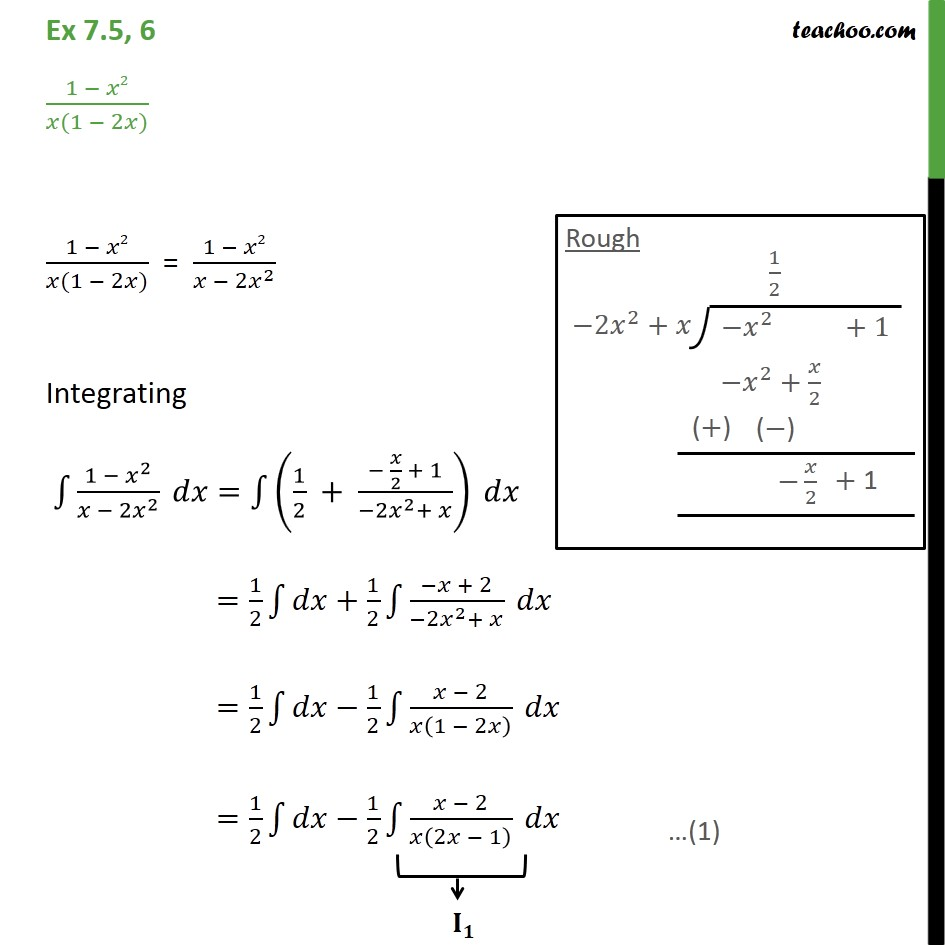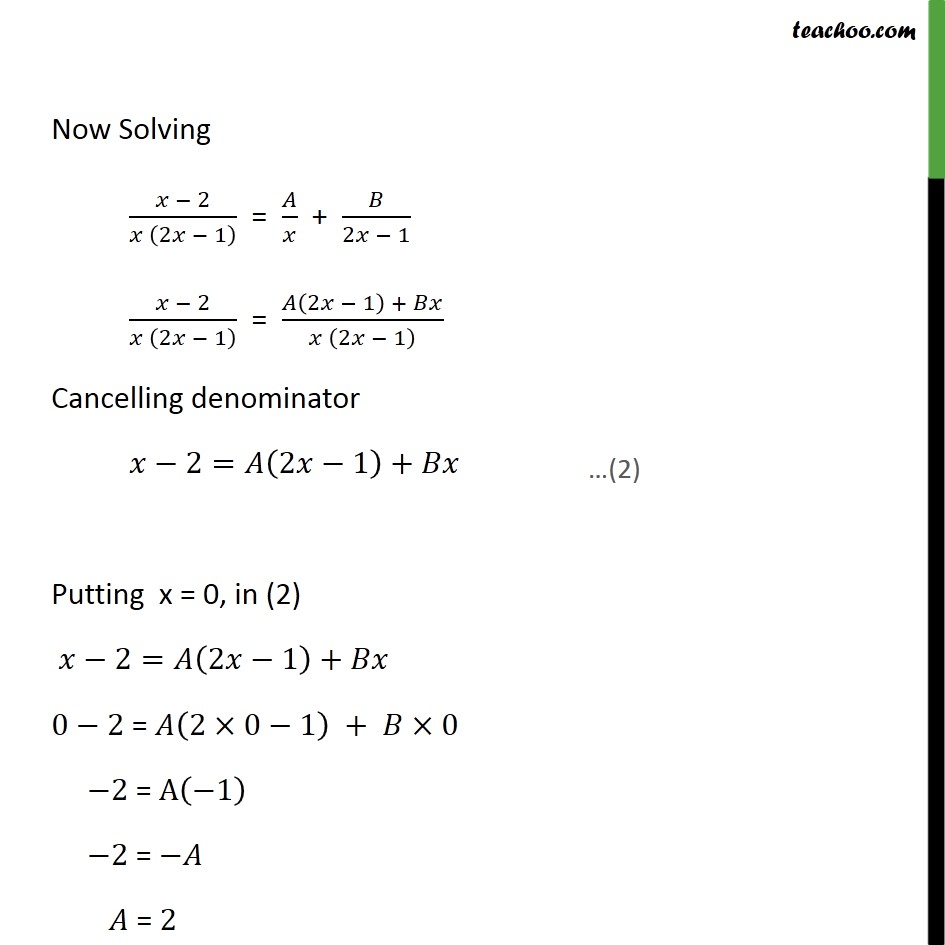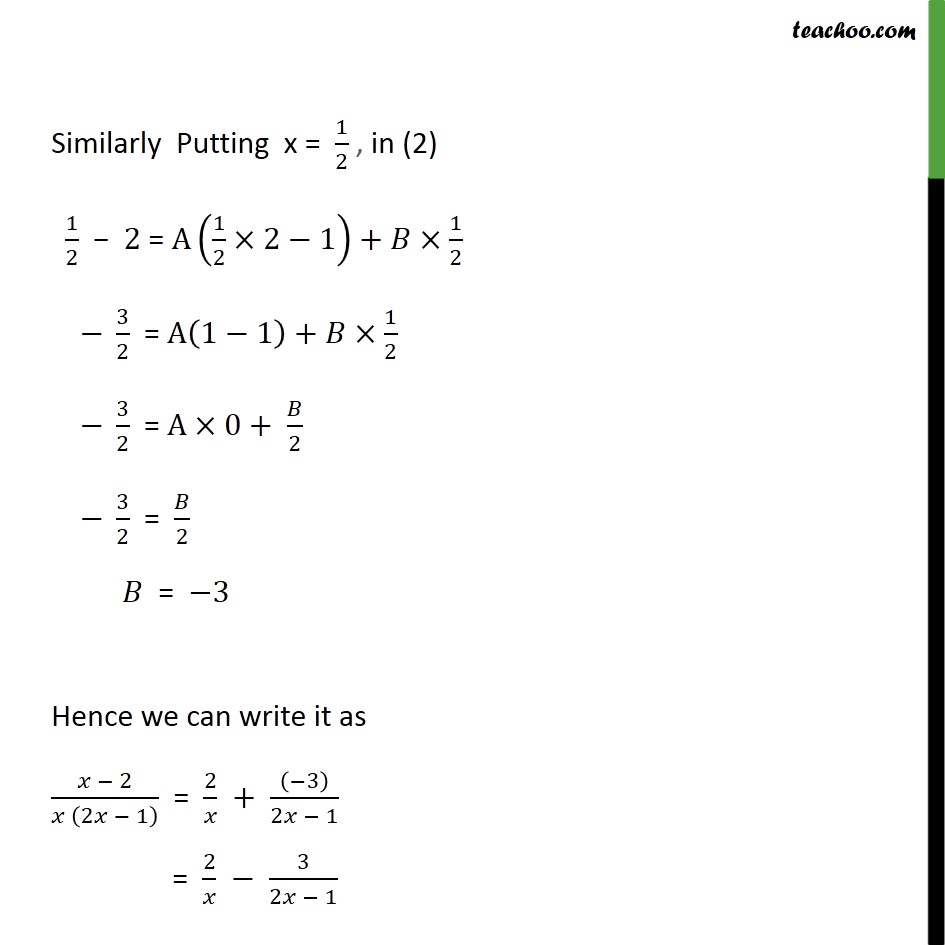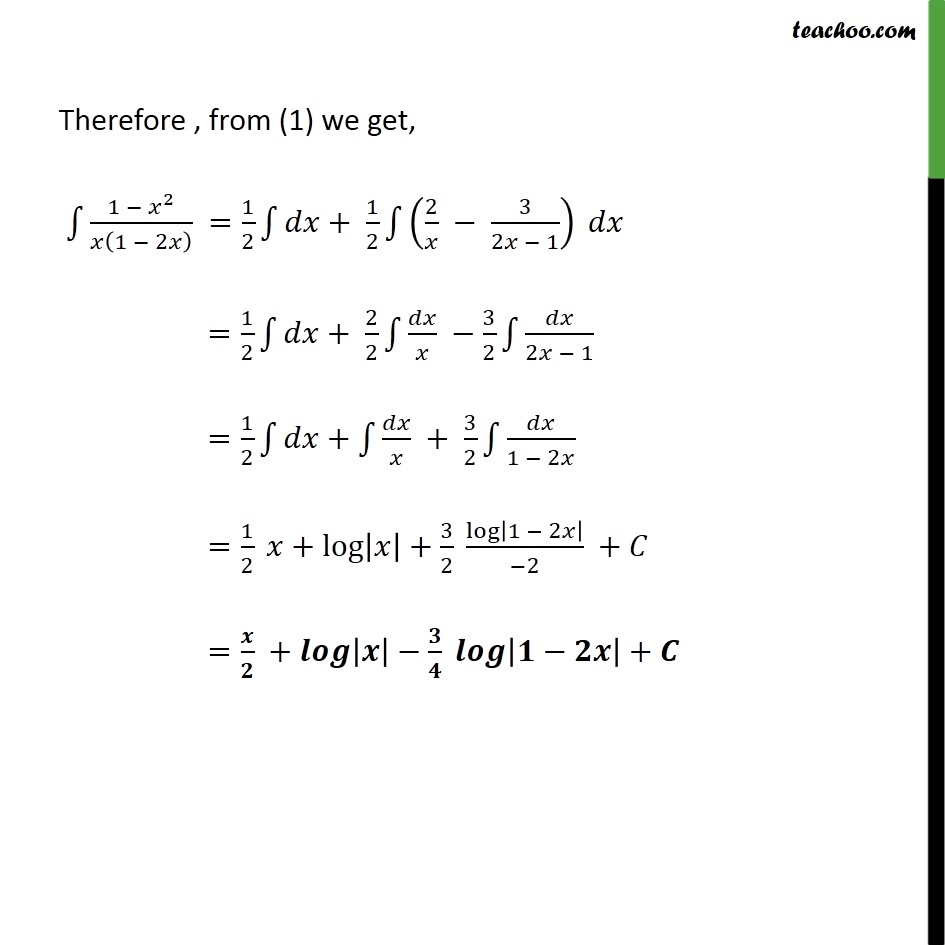1. Chapter 7 Class 12 Integrals
2. Serial order wise
3. Ex 7.5

Transcript

Ex 7.5, 6 (1 − 𝑥2)/(𝑥(1 − 2𝑥)) (1 − 𝑥2)/(𝑥(1 − 2𝑥)) = (1 − 𝑥2)/(𝑥 − 2𝑥^2 ) Integrating ∫1▒(1 − 𝑥^2)/(𝑥 − 2𝑥^2 ) 𝑑𝑥=∫1▒(1/2 + (− 𝑥/2 + 1)/(−2𝑥^2+ 𝑥)) 𝑑𝑥 =1/2 ∫1▒𝑑𝑥+1/2 ∫1▒(−𝑥 + 2)/(−2𝑥^2+ 𝑥) 𝑑𝑥 =1/2 ∫1▒𝑑𝑥−1/2 ∫1▒(𝑥 − 2)/𝑥(1 − 2𝑥) 𝑑𝑥 =1/2 ∫1▒𝑑𝑥−1/2 ∫1▒(𝑥 − 2)/𝑥(2𝑥 − 1) 𝑑𝑥 Now Solving (𝑥 − 2)/(𝑥 (2𝑥 − 1) ) = 𝐴/𝑥 + 𝐵/(2𝑥 − 1) (𝑥 − 2)/(𝑥 (2𝑥 − 1) ) = (𝐴(2𝑥 − 1) + 𝐵𝑥)/(𝑥 (2𝑥 − 1) ) Cancelling denominator 𝑥−2=𝐴(2𝑥−1)+𝐵𝑥 Putting x = 0, in (2) 𝑥−2=𝐴(2𝑥−1)+𝐵𝑥 0−2 = 𝐴(2×0−1) + 𝐵×0 −2 = A(−1) −2 = −𝐴 𝐴 = 2 Similarly Putting x = 1/2 , in (2) 1/2 − 2 = A(1/2×2−1)+𝐵×1/2 − 3/2 = A(1−1)+𝐵×1/2 − 3/2 = A×0+ 𝐵/2 − 3/2 = 𝐵/2 𝐵 = −3 Hence we can write it as (𝑥 − 2)/(𝑥 (2𝑥 − 1) ) = 2/𝑥 + ((−3))/(2𝑥 − 1) = 2/𝑥 − 3/(2𝑥 − 1) Therefore , from (1) we get, ∫1▒(1 − 𝑥^2)/𝑥(1 − 2𝑥) =1/2 ∫1▒𝑑𝑥+ 1/2 ∫1▒(2/𝑥 − 3/(2𝑥 − 1)) 𝑑𝑥 =1/2 ∫1▒𝑑𝑥+ 2/2 ∫1▒〖𝑑𝑥/𝑥 −3/2〗 ∫1▒𝑑𝑥/(2𝑥 − 1) =1/2 ∫1▒𝑑𝑥+∫1▒〖𝑑𝑥/𝑥 + 3/2〗 ∫1▒𝑑𝑥/(1 − 2𝑥) =1/2 𝑥+log⁡|𝑥|+3/2 log⁡|1 − 2𝑥|/(−2) +𝐶 =𝒙/𝟐 +𝒍𝒐𝒈⁡|𝒙|−𝟑/𝟒 𝒍𝒐𝒈⁡|𝟏−𝟐𝒙|+𝑪

Ex 7.5

Chapter 7 Class 12 Integrals
Serial order wise